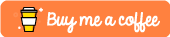# Functions in Swift - Introduction

This post is going to explain what a function is and how to use it. It is a basic look at the parts of a function. More detail will be given in other posts.

# What is a Function

According to the Swift documentation, “functions are self-contained chunks of code that perform a specific task.”

Usually a function is defined when the task needs to be repeated or used somewhere else.

# How to define a function

When defining a function, it needs to start with `func` . This tells the swift compiler that the next chunk of code is going to be a function. Then it needs an argument name followed by parentheses. Inside the parentheses, is where parameters go.

Function parameters are not required for a function. Parameters are necessary when the task needs data not accessible by the function. All parameters in a function must have a unique name. When using the parameters in the function, the parameter name is used to access the value it contains.

After the parameters are defined, a return type needs to be set if the function is returning a value. Not all functions need to return a value, so this step is optional. If declaring a return value, then the function must have a return statement in it. If not, then the compiler will throw an error.

Below are three examples of functions, one without parameters and no return value, one with parameters and no return value, and the last one has parameters and a return value.

## Example of a Function without Parameters and no Return Value

``````func add(){
let a: Int = 5
let b: Int = 10
let ans: Int = a + b
print(ans)
}

add() // this is executing the function
``````

In this example, any time `add()` is called it will always print 15 since 5 + 10 = 15.

## Example of a Function with Parameters and no Return Value

``````func add(numA: Int, numB: Int) {
let ans: Int = numA + numB
print(ans)
}
``````

In order to use `add(numA:numB)` , then two integers must be passed to it, and then it will print the sum of the two integers. The code below shows how to call the function and use parameters.

``````add(numA: 1, numB: 10) // Prints 11
add(numA: 15, numB: 10) // Prints 25
``````

## Example of a Function with Parameters and a Return Value

``````func sub(numA: Int, numB: Int) -> Int {
let ans: Int = numA - numB
return ans
}
``````

Function `sub(numA:numB)` will subtract `numB` from `numA` and then return the answer in order for the answer to be used somewhere else.

``````print(sub(numA: 10, numB: 5)) // Prints 5

var ans1 = sub(numA: 10, numB: 5) // Stores 5 in ans1
var ans2 = sub(numA: 12, numB: 6) // Stores 6 in ans2
print(sub(numA: ans2, numB: ans1)) // Prints 1
``````

Above, the first line prints the answer from `sub(numA: 10, numB: 5)` . The next three lines then store two calls to `sub(numA:numB)` and prints the answer to the last one.

If you enjoyed this post and want more, please consider subscribing to my Patreon and checking out my other posts!

If you don't like having more subscriptions, consider buying me a coffee by clicking the image below!This post is also available on DEV.